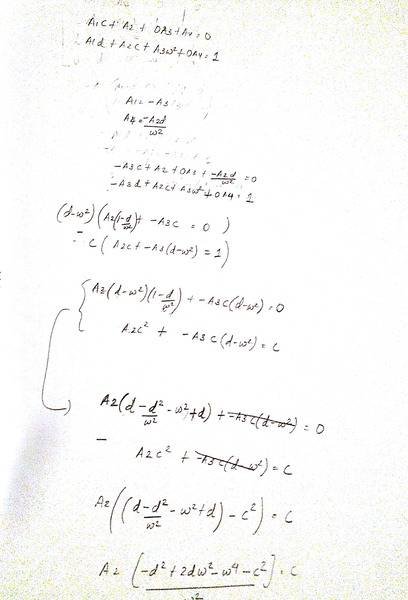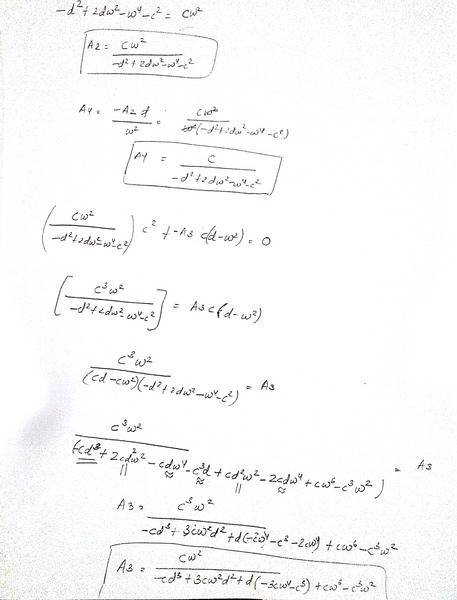# Solve this system of linear equations

• JaZZyCooL

## Homework Statement

Solve the following system of equations

## Homework Equations

A1 + A3 = 0
cA1 + A2 + A4 = 0
dA1 + cA2 + w^2 A3 = 1
dA2 + w^2A4 = 0

## The Attempt at a Solution

https://imgur.com/YSG5oGN - Page 1
https://imgur.com/dGbccsE - Page 2

I checked with Mathematica. Your expressions for A2 and A4 are correct. Your expression for A3 is not.

•scottdave
I checked with Mathematica. Your expressions for A2 and A4 are correct. Your expression for A3 is not.
Knowing that, the OP should be able to easily fix A1 and A3.

Knowing that, the OP should be able to easily fix A1 and A3.
I agree. This particular system lends itself to using Cramer's rule because of all the zeroes on the right hand side.

## Homework Statement

Solve the following system of equations

## Homework Equations

A1 + A3 = 0
cA1 + A2 + A4 = 0
dA1 + cA2 + w^2 A3 = 1
dA2 + w^2A4 = 0

## The Attempt at a Solution

https://imgur.com/YSG5oGN - Page 1
https://imgur.com/dGbccsE - Page 2#### Attachments

the system shows two nonlinear equations. Not linear system.

the system shows two nonlinear equations. Not linear system.
The system is linear in A1, A2, A3, and A4 .

The system is linear in A1, A2, A3, and A4 .
These two equations are shown in the system:
dA1 + cA2 + w^2 A3 = 1
dA2 + w^2A4 = 0
Not linear. That shows an exponent of 2 on the w. If I misunderstand, then w is not a variable; but A1, A2, and A3 must be the variables. If this latter is the case, then yes; linear system.